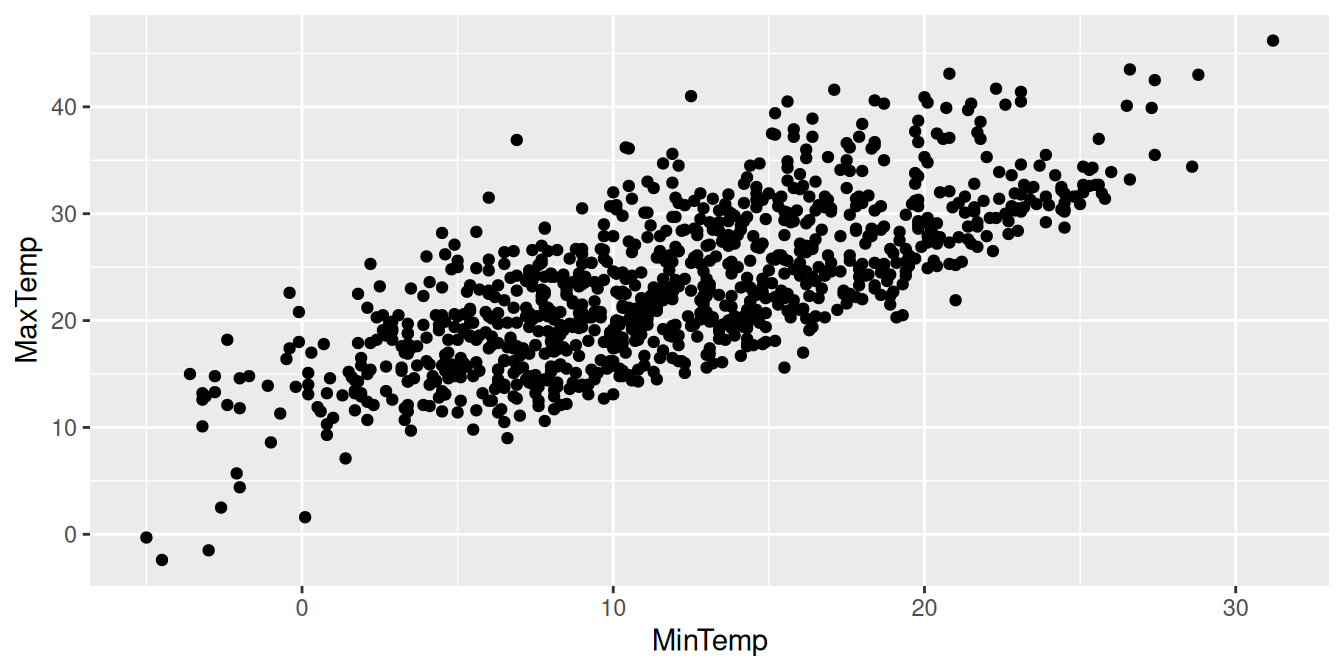## 11.53 Scatter Plot

20220111set.seed(26439)
ds %>%
sample_n(1000) %>%
ggplot(aes(x=min_temp, y=max_temp)) +
geom_point() +
labs(x=vnames["min_temp"],
y=vnames["max_temp"])

The simplest of plots is a scatter plot which displays a two dimensional plot of points. The dimensions, specified through the aesthetics function ggplot2::aes(), are the x= and y= axes. The observations from the dataset, the rows, are plotted, scattering the points over the plot. Even with a simple plot we can observe a generally linear relationship between the two variables. That is, higher values of minimum temperature are loosely correlated with higher values of maximum temperature.

Scattering too many points (thousands or more) over a plot can result in a loss of information as the plot ends up mostly black with points overlaying other points. Some solutions are presented in this chapter to this problem, but for illustration here we randomly choose just 1,000 points. A random number seed is fixed using base::set.seed() so that each time we do the random sample we get the same random sample, again for illustration.

The template dataset variable ds (having 226,868 observations/rows) is sampled using dplyr::sample_n(). This subset of 1,000 observations is piped (%>%) into ggplot2::ggplot(). The aesthetics ggplot2::aes() are set up with min_temp as the x= axis and max_temp as the y= axis. The points (observations) are added to the plot using ggplot2::geom_point().

For presentation the x and y axis are labelled with the original names of the variables, using ggplot2::labs().

Your donation will support ongoing availability and give you access to the PDF version of this book. Desktop Survival Guides include Data Science, GNU/Linux, and MLHub. Books available on Amazon include Data Mining with Rattle and Essentials of Data Science. Popular open source software includes rattle, wajig, and mlhub. Hosted by Togaware, a pioneer of free and open source software since 1984. Copyright © 1995-2022 Graham.Williams@togaware.com Creative Commons Attribution-ShareAlike 4.0